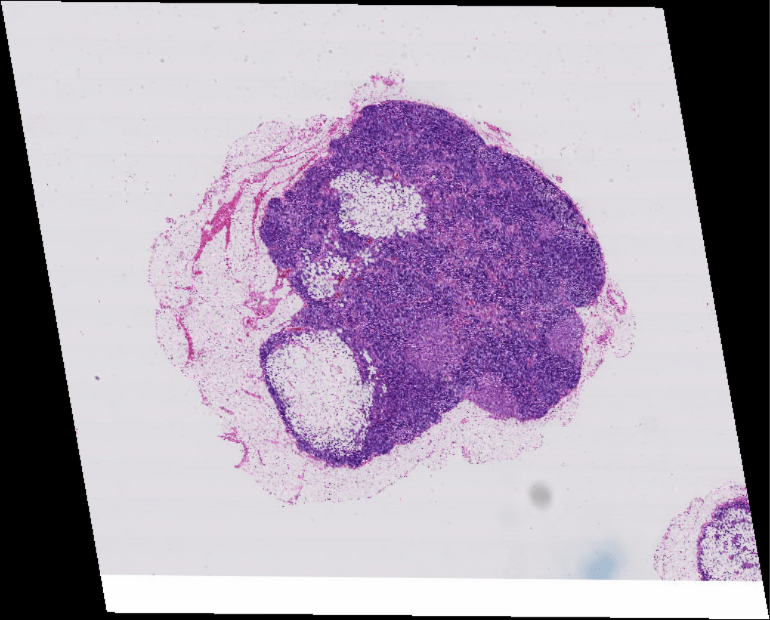# Warp Blocked Image at Coarse and Fine Resolution Levels

This example shows how to apply a geometric transformation (warping) to a blocked image.

Applying a geometric transformation to an image is a key step in many image processing applications like image registration. You can use `imwarp` to warp coarse images that fit in memory. For large, high-resolution images that do not fit in memory, use a blocked image. Set the spatial referencing of the warped image to preserve characteristics of the image such as pixel extents.

Create a blocked image using a modified version of image "tumor_091.tif" from the CAMELYON16 data set. The original image is a training image of a lymph node containing tumor tissue. The original image has eight resolution levels, and the finest level has resolution 53760-by-61440. The modified image has only three coarse resolution levels. The spatial referencing of the modified image has been adjusted to enforce a consistent aspect ratio and to register features at each level.

`bim = blockedImage('tumor_091R.tif');`

### Apply Geometric Transformation to Coarse Image

Create an `affine2d` object that stores information about an affine geometric transformation. This transformation applies translation and shear.

```tform = affine2d([ 0.99 0.01 0 0.17 0.98 0 120 -30 1]);```

Get the image at the coarsest resolution level.

`imCoarse = gather(bim);`

Warp the coarse image by using `imwarp`. Display the original image and the warped image in a montage.

```imCoarseWarped = imwarp(imCoarse,tform); figure imshow(imCoarseWarped)```### Create Spatial Referencing for Warped Fine Image

Before applying the geometric transformation to the image at a fine resolution level, calculate the spatial referencing of the blocked image after the warping. Use this spatial referencing when transforming blocks

Get the pixel extent of the original image from its spatial referencing information.

`inPixelExtent = (bim.WorldEnd(1,:)-bim.WorldStart(1,:))./bim.Size(1,:);`

Calculate the output horizontal and vertical spatial limits when the transformation is applied.

```yWorldLimits = [bim.WorldStart(1,1), bim.WorldEnd(1,1)]; xWorldLimits = [bim.WorldStart(1,2), bim.WorldEnd(1,2)]; [xout, yout] = outputLimits(tform,xWorldLimits,yWorldLimits);```

Calculate the size of the output image that preserves the pixel extent. Specify the image size in the format [numrows, numcols].

```outImgSize = [ceil(diff(yout)/inPixelExtent(1)),... ceil(diff(xout)/inPixelExtent(2))];```

Store the spatial referencing information of the warped image. Set the world limits and image size of the warped image.

```outWorldStart = [yout(1),xout(1)]; outWorldEnd = [yout(2), xout(2)];```

Calculate the corresponding output pixel dimensions.

```outPixelExtent = (outWorldEnd-outWorldStart)./outImgSize; halfPixWidth = outPixelExtent/2;```

### Apply Block-Wise Warping to Fine Image

Create a writable blocked image by specifying the output spatial referencing information. Specify a block size that is large enough to use memory efficiently.

```outBlockSize = [1024 1024 3]; bwarped = blockedImage([],[outImgSize 3],outBlockSize,uint8(0),... 'Mode','w',... 'WorldStart', [yout(1), xout(1)],... 'WorldEnd', [yout(2), xout(2)]);```

Loop through the output image, one block at a time. For each output block:

1. Find the coordinates of the four corners of the output block.

2. Inverse map these coordinates back to the input to get the input (source) region.

3. Read the contents of the input region.

4. Create spatial referencing describing the input region.

5. Calculate the output block content by using `imwarp`.

6. Write the output block to the output image by using the `setBlock` function.

If you have Parallel Computing Toolbox™, then you can replace the outer `for` statement with a `parfor` statement to run the loop in parallel.

```inYWorldLimits = [bim.WorldStart(1,1), bim.WorldEnd(1,1)]; inXWorldLimits = [bim.WorldStart(1,2), bim.WorldEnd(1,2)]; for rBlockInd = 1:bwarped.SizeInBlocks(1) for cBlockInd = 1:bwarped.SizeInBlocks(2) blockSub = [rBlockInd, cBlockInd 1]; % Center of top left pixel of this block in world units blockStartSub = blocksub2sub(bwarped, blockSub); blockStartWorld = sub2world(bwarped, blockStartSub); % Center of bottom right pixel of this block in world units blockEndSub = blockStartSub + outBlockSize - 1; blockEndWorld = sub2world(bwarped, blockEndSub); % Spatial reference which describes this block (Note: spatial % referencing is in x-y order, while blockStart etc are in y-x % order). Ensure to move the region outwards by half a pixel to % reference the outer edge of this block. outRegionRef = imref2d(fliplr(outBlockSize(1:2))); outRegionRef.YWorldLimits = [blockStartWorld(1)-halfPixWidth(1),... blockEndWorld(1)+halfPixWidth(1)]; outRegionRef.XWorldLimits = [blockStartWorld(2)-halfPixWidth(2),... blockEndWorld(2)+halfPixWidth(2)]; % Output bounding box in world coordinates outbbox = [ fliplr(blockStartWorld(1:2)) blockStartWorld(2) blockEndWorld(1) blockEndWorld(2) blockStartWorld(1) fliplr(blockEndWorld(1:2))]; % Get corresponding input region. Note: This region need NOT be % rectangular if the transformation includes shear inRegion = transformPointsInverse(tform,outbbox); % Clamp region to image extents inRegion(:,2) = max(inRegion(:,2),inYWorldLimits(1)); inRegion(:,2) = min(inRegion(:,2),inYWorldLimits(2)); inRegion(:,1) = max(inRegion(:,1),inXWorldLimits(1)); inRegion(:,1) = min(inRegion(:,1),inXWorldLimits(2)); % Find the corresponding input bounding box inbboxStart = [min(inRegion(:,1)) min(inRegion(:,2))]; inbboxEnd = [max(inRegion(:,1)) max(inRegion(:,2))]; % Move to y-x (row-col) order inbboxStart = fliplr(inbboxStart); inbboxEnd = fliplr(inbboxEnd); % Convert to pixel subscripts inbboxStartSub = world2sub(bim, [inbboxStart 1]); inbboxEndSub = world2sub(bim, [inbboxEnd 3]); % Read corresponding input region inputRegion = getRegion(bim,inbboxStartSub,inbboxEndSub); % Input region's spatial referencing inRegionRef = imref2d(size(inputRegion)); % Convert the actual region pixel's centers back to world % coordinates. inbboxStart = sub2world(bim, inbboxStartSub); inbboxEnd = sub2world(bim, inbboxEndSub); % Convert to pixel edges from pixel centers inRegionRef.YWorldLimits = [inbboxStart(1)-halfPixWidth(1),... inbboxEnd(1)+halfPixWidth(2)]; inRegionRef.XWorldLimits = [inbboxStart(2)-halfPixWidth(1),... inbboxEnd(2)+halfPixWidth(2)]; % Warp this block warpedBlock = imwarp(inputRegion,inRegionRef,tform,'OutputView',outRegionRef); % Set the block data in the output blocked image setBlock(bwarped,blockSub,warpedBlock); end end```

Display the warped image.

```bwarped.Mode = 'r'; figure bigimageshow(bwarped)```